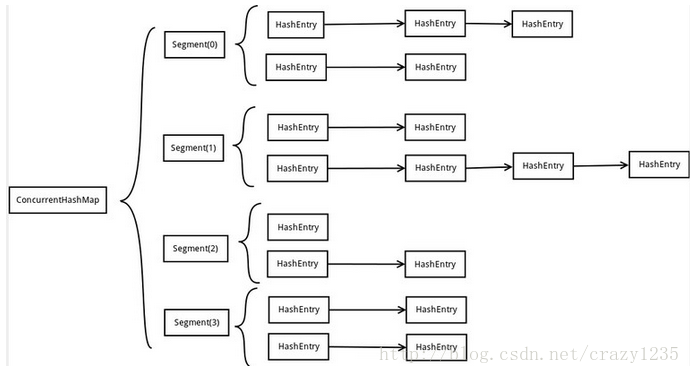ConcurrentHashMap介绍

ConcurrnetHashMap是HashMap线程安全版本的实现，阅读本文的朋友，我先假定您对HashMap的使用已经非常的了解了，如果不太熟悉HashMap实现的读者，可以先对其进行一下了解，这样可以帮助您更好的理解ConcurrentHashMap。

ConcurrentHashMap是在Java 5版本中加入的，在此之前，由于HashMap的实现是线程不安全的，因此使用的时候，需要的额外的同步工作，Java 5以后，在多线程操作的场景下，就可以直接使用ConcurrentHashMap来满足线程安全的要求。

ConcurrentHashMap在1.8的版本之前的实现与1.8中实现差异较大，在1.8版本后进行了较大的重构，由于在JDK 1.8版本后的设计非常复杂，因此本篇，我们依旧基于JDK 1.7的版本进行分析。ConcurrentHashMap的数据存储结构与HashMap很相似，底层都采用了一个Table数组来存储数据，关于拉链表的存储模式，这里不再赘述， 如果不太清楚的读者，可以去了解一下HashMap的实现，ConcurrentHashMap中引入了Segment的段级锁的概念，利用段级锁来实现细粒度的并发控制，这块我们后面会详细来说。

ConcurrentHashMap 结构

ConcurrentHashMap 继承了AbstractMap，实现了ConcurrentMap，同时间接实现了Map接口，首先我们来看一下其比较主要的属性：

主要属性：

// 默认数据初始化大小，可以通过构造函数指定
static final int DEFAULT_INITIAL_CAPACITY = 16;

//默认加载因子，可以通过构造函数指定
static final float DEFAULT_LOAD_FACTOR = 0.75f;

//默认并发等级，可以通过构造函数指定
static final int DEFAULT_CONCURRENCY_LEVEL = 16;

//最大容量，2的30次幂
static final int MAXIMUM_CAPACITY = 1 << 30;

//segments段级锁数组
final Segment<K,V>[] segments;

//key集合
transient Set<K> keySet;

//元素集合
transient Set<Map.Entry<K,V>> entrySet;

//value集合
transient Collection<V> values;


HashEntry：

static final class HashEntry<K,V> {
final int hash;
final K key;
volatile V value;
volatile HashEntry<K,V> next;

HashEntry(int hash, K key, V value, HashEntry<K,V> next) {
this.hash = hash;
this.key = key;
this.value = value;
this.next = next;
}

/**
* Sets next field with volatile write semantics.
* (See above about use of putOrderedObject.)
*/
final void setNext(HashEntry<K,V> n) {
UNSAFE.putOrderedObject(this, nextOffset, n);
}

// Unsafe mechanics
static final sun.misc.Unsafe UNSAFE;
static final long nextOffset;
static {
try {
UNSAFE = sun.misc.Unsafe.getUnsafe();
Class k = HashEntry.class;
nextOffset = UNSAFE.objectFieldOffset
(k.getDeclaredField("next"));
} catch (Exception e) {
throw new Error(e);
}
}
}


HashEntry是ConcurrentHashMap的一个内部类，其结构与HashMap的Entry基本一致，不同的是setNext()方法，这个方法可以保证写入后，可以立即对其他线程可见，通过native方法实现了voliate的效果。

Segment：

static final class Segment<K,V> extends ReentrantLock implements Serializable {

private static final long serialVersionUID = 2249069246763182397L;

static final int MAX_SCAN_RETRIES =
Runtime.getRuntime().availableProcessors() > 1 ? 64 : 1;

transient volatile HashEntry<K,V>[] table;

transient int count;

transient int modCount;

transient int threshold;

Segment(float lf, int threshold, HashEntry<K,V>[] tab) {
this.threshold = threshold;
this.table = tab;
}
//......
//省略具体方法实现
//......
}


ConcurrentHashMap构造初始化

public ConcurrentHashMap(int initialCapacity,
if (!(loadFactor > 0) || initialCapacity < 0 || concurrencyLevel <= 0)
throw new IllegalArgumentException();
//最大大小为65535
if (concurrencyLevel > MAX_SEGMENTS)
concurrencyLevel = MAX_SEGMENTS;
// Find power-of-two sizes best matching arguments
int sshift = 0;
int ssize = 1;
while (ssize < concurrencyLevel) {
++sshift;
ssize <<= 1;
}
this.segmentShift = 32 - sshift;
if (initialCapacity > MAXIMUM_CAPACITY)
initialCapacity = MAXIMUM_CAPACITY;
int c = initialCapacity / ssize;
if (c * ssize < initialCapacity)
++c;
int cap = MIN_SEGMENT_TABLE_CAPACITY;
while (cap < c)
cap <<= 1;
// create segments and segments
Segment<K,V> s0 =
(HashEntry<K,V>[])new HashEntry[cap]);
Segment<K,V>[] ss = (Segment<K,V>[])new Segment[ssize];
UNSAFE.putOrderedObject(ss, SBASE, s0); // ordered write of segments
this.segments = ss;
}


HashEntry的初始化大小根据initialCapacity计算得来，默认值为2。

ConcurrentHashMap put()实现

put():

public V put(K key, V value) {
Segment<K,V> s;
if (value == null)
throw new NullPointerException();
int hash = hash(key);
int j = (hash >>> segmentShift) & segmentMask;
if ((s = (Segment<K,V>)UNSAFE.getObject          // nonvolatile; recheck
(segments, (j << SSHIFT) + SBASE)) == null) //  in ensureSegment
s = ensureSegment(j);
return s.put(key, hash, value, false);
}


put()中主要分为几步操作：

1、判断值是否为null

2、计算hash值

3、定位要存储到哪个Segment，如果不存在，则进行创建

4、调用Segment的put()方法存储元素

Segment put():

final V put(K key, int hash, V value, boolean onlyIfAbsent) {
HashEntry<K,V> node = tryLock() ? null :
scanAndLockForPut(key, hash, value);
V oldValue;
try {
HashEntry<K,V>[] tab = table;
int index = (tab.length - 1) & hash;
HashEntry<K,V> first = entryAt(tab, index);
for (HashEntry<K,V> e = first;;) {
if (e != null) {
K k;
if ((k = e.key) == key ||
(e.hash == hash && key.equals(k))) {
oldValue = e.value;
if (!onlyIfAbsent) {
e.value = value;
++modCount;
}
break;
}
e = e.next;
}
else {
if (node != null)
node.setNext(first);
else
node = new HashEntry<K,V>(hash, key, value, first);
int c = count + 1;
if (c > threshold && tab.length < MAXIMUM_CAPACITY)
rehash(node);
else
setEntryAt(tab, index, node);
++modCount;
count = c;
oldValue = null;
break;
}
}
} finally {
unlock();
}
return oldValue;
}


1、尝试获取锁

2、定位HashEntry[]数组中元素要存储的位置

3、遍历链表，查找key是否已经存在，如果存在，判断onlyIfAbsent的值，决定是否要对旧值进行覆盖，默认情况下会进行覆盖，并返回旧值

4、如果key不存在，将元素放入数组指定位置的拉链表的首节点

5、释放锁

ConcurrentHashMap get()实现

get()

public V get(Object key) {
Segment<K,V> s; // manually integrate access methods to reduce overhead
HashEntry<K,V>[] tab;
int h = hash(key);
long u = (((h >>> segmentShift) & segmentMask) << SSHIFT) + SBASE;
if ((s = (Segment<K,V>)UNSAFE.getObjectVolatile(segments, u)) != null &&
(tab = s.table) != null) {
for (HashEntry<K,V> e = (HashEntry<K,V>) UNSAFE.getObjectVolatile
(tab, ((long)(((tab.length - 1) & h)) << TSHIFT) + TBASE);
e != null; e = e.next) {
K k;
if ((k = e.key) == key || (e.hash == h && key.equals(k)))
return e.value;
}
}
return null;
}


get()的操作相对比较简单，主要分为下面几个步骤：

1、计算key的hash值

2、定位到存储的Segment，再定位到存储数组中的具体位置

3、遍历拉链表，查找key值

4、找到对应的key，返回value，否则返回null

get()方法的实现中，我们可以发现一点，没有一处使用到锁的地方，为什么get()可以支持并发操作下不使用锁呢？

ConcurrentHashMap size()实现

size()

public int size() {
// Try a few times to get accurate count. On failure due to
// continuous async changes in table, resort to locking.
final Segment<K,V>[] segments = this.segments;
int size;
boolean overflow; // true if size overflows 32 bits
long sum;         // sum of modCounts
long last = 0L;   // previous sum
int retries = -1; // first iteration isn't retry
try {
for (;;) {
if (retries++ == RETRIES_BEFORE_LOCK) {
for (int j = 0; j < segments.length; ++j)
ensureSegment(j).lock(); // force creation
}
sum = 0L;
size = 0;
overflow = false;
for (int j = 0; j < segments.length; ++j) {
Segment<K,V> seg = segmentAt(segments, j);
if (seg != null) {
sum += seg.modCount;
int c = seg.count;
if (c < 0 || (size += c) < 0)
overflow = true;
}
}
if (sum == last)
break;
last = sum;
}
} finally {
if (retries > RETRIES_BEFORE_LOCK) {
for (int j = 0; j < segments.length; ++j)
segmentAt(segments, j).unlock();
}
}
return overflow ? Integer.MAX_VALUE : size;
}


ConcurrentHashMap的做法比较精妙，首先会尝试三次不加锁计算，如果过程中ConcurrentHashMap的元素没有被修改，那么说明count值是准确的，可以直接返回；如果三次都失败，计算过程中元素数都发生了修改，那么再进行加锁操作，重新统计。这样可以保证效率的最大化，因为修改操作与size()操作同时发生的概率并不是很大。

总结

1、ConcurrentHashMap是线程安全版本的HashMap，采用Segment段级锁的机制进行实现，Segment继承于ReentrantLock。

2、ConcurrentHashMap中的key和value值都不能为null，HashMap中key可以为null，HashTable中key不能为null。

3、ConcurrentHashMap是线程安全的类并不能保证使用了ConcurrentHashMap的操作都是线程安全的。

ConcurrentHashMap的实现依赖于ReentrantLock，如果对ReentrantLock不了解的读者，可以查看之前的文章对于ReentrantLock的介绍。